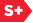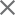### Proceedings Paper

Adaptive subspace decomposition for constrained nonlinear inversion of linear inverse problems
Author(s): Tim E. Olson
Format Member Price Non-Member Price
PDF \$17.00 \$21.00

Paper Abstract

Many problems in applied mathematics involve recovering a function f from measurements of Lf, where L is a known operator. If L is a linear operator with a bounded inverse, then f can recovered from noisy data Lf + n via standard techniques with little difficulty. The recovery of f from noisy data Lf + n is much more difficult if L is an operator whose inverse is unbounded. One such problem is the recovery of a function f : R2 —R, from limited knowledge of its Fourier transform f. Thus we are interested in reconstructing f from Lf where L is an operator which reflects the limited knowledge of the Fourier transform of f. This problem is motivated by the limited angle tomography problem. This recovery problem is ill-posed and unsolvable without a priori assumptions about f. With proper a priori knowledge, however, this problem is solvable. For example, if f is compactly supported, then f will be an analytic function, and therefore f will be uniquely determined by its values on any region containing a limit point . Classical analytic continuation techniques are not numerically feasible  since the operator L will generally not have a bounded inverse. A technique for doing analytic continuation was introduced by Papoulis in . This algorithm depends upon the eigenfunctions of the continuous time and band-limiting operators, which were extensively studied in the classical works of Slepian, Pollack, and Landau . The convergence of the algorithm is dependent upon the eigenvalues of the joint time- and band-limiting operators, and except in extreme cases, this algorithm is not feasible numerically . We will model these data recovery problems as the inversion of a compact operator L ( for limited data ); where Lf = Xs(Xcj), S is the common support of the functions under consideration, C is the set where we can measure the Fourier transform of f, and Xs is a characteristic, or indicator function on the set S. One tool for analyzing the spectra of these discrete approximations to L will be the theory of finite Toeplitz forms, originally introduced by Siegö . We will show that the study of these finite Toeplitz forms will give us some clues concerning the construction of an accurate, stable inversion for L, even when the continuous spectra of L suggests that it is not invertible. Possibly more importantly, we will examine the addition of nonlinear constraints into this problem in order for further mollification

Paper Details

Date Published: 1 September 1995
PDF: 14 pages
Proc. SPIE 2569, Wavelet Applications in Signal and Image Processing III, (1 September 1995); doi: 10.1117/12.217620
Show Author Affiliations
Tim E. Olson, Dartmouth College (United States)

Published in SPIE Proceedings Vol. 2569:
Wavelet Applications in Signal and Image Processing III
Andrew F. Laine; Michael A. Unser, Editor(s)